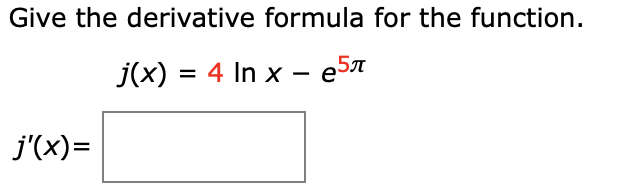Give the derivative formula for the function.j(x) 4 In x e5=j(x)

Questionhelp_outlineImage TranscriptioncloseGive the derivative formula for the function. j(x) 4 In x e5 = j(x) fullscreen
Step 1

The function is ...

Want to see the full answer?

See Solution

Want to see this answer and more?

Our solutions are written by experts, many with advanced degrees, and available 24/7

See Solution
Tagged in

Derivative